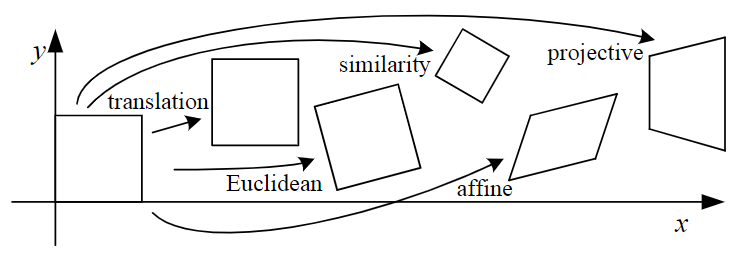写在前面

2D图像常见的坐标变换如下图所示：Basic set of 2D planar transformations

变换矩阵形式

$$\left[ \begin{array}{l}{x’} \\ {y’}\end{array}\right]=\left[ \begin{array}{ll}{a} & {b} \\ {c} & {d}\end{array}\right] \left[ \begin{array}{l}{x} \\ {y}\end{array}\right]$$

$$\left[ \begin{array}{l}{x^{\prime}} \\ {y^{\prime}} \\ {1}\end{array}\right] =\left[ \begin{array}{lll}{a} & {b} & {c}\\ {d} & {e} & {f} \end{array}\right] \left[ \begin{array}{l}{x} \\ {y} \\ {1}\end{array}\right]$$

$$\left[ \begin{array}{ccc}{\cos (\theta)} & {-\sin (\theta)} & {t_{x}} \\ {\sin (\theta)} & {\cos (\theta)} & {t_{y}}\end{array}\right] \left[ \begin{array}{l}{x} \\ {y} \\ {1}\end{array}\right]=\left[ \begin{array}{c}{x^{\prime}} \\ {y^{\prime}} \\ {1}\end{array}\right]$$

$$\left[ \begin{array}{ccc}{s\cos (\theta)} & {-s\sin (\theta)} & {t_{x}} \\ {s\sin (\theta)} & {s\cos (\theta)} & {t_{y}}\end{array}\right] \left[ \begin{array}{l}{x} \\ {y} \\ {1}\end{array}\right]=\left[ \begin{array}{c}{x^{\prime}} \\ {y^{\prime}} \\ {1}\end{array}\right]$$

变换矩阵的理解与记忆

$$\left[ \begin{array}{l}{x} \\ {y}\end{array}\right] = x\left[ \begin{array}{l}{1} \\ {0}\end{array}\right] + y\left[ \begin{array}{l}{0} \\ {1}\end{array}\right] = \left[ \begin{array}{ll}{1} & {0} \\ {0} & {1}\end{array}\right] \left[ \begin{array}{l}{x} \\ {y}\end{array}\right]$$

$$\left[ \begin{array}{l}{x’} \\ {y’}\end{array}\right] = x\left[ \begin{array}{l}{\cos (\theta)} \\ { \sin (\theta)}\end{array}\right] + y\left[ \begin{array}{r}{- \sin (\theta)} \\ { \cos (\theta)}\end{array}\right] = \left[ \begin{array}{lr}{\cos (\theta)} & {-\sin (\theta)} \\ {\sin (\theta)} & {\cos (\theta)}\end{array}\right] \left[ \begin{array}{l}{x} \\ {y}\end{array}\right]$$

• 所有变换矩阵只需关注一点：坐标系的变化，即基向量和原点的变化
• 坐标系变化到哪里，坐标系中的所有点也跟着做同样的变化
• 坐标系的变换分为 基向量的变化 以及 坐标原点的变化，在仿射变换矩阵 $\left[ \begin{array}{lll}{a} & {b} & {c}\\ {d} & {e} & {f} \\ 0 & {0} & {1}\end{array}\right]$中， $\left[ \begin{array}{l}{a} \\ {d}\end{array}\right]$和$\left[ \begin{array}{l}{b} \\ {e}\end{array}\right]$为新的基向量，$\left[ \begin{array}{l}{c} \\ {f}\end{array}\right]$为新的坐标原点，先变化基向量，再变化坐标原点；